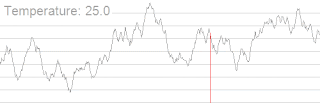# Complete Guide for Simulated Annealing | Python Implementation

In this article, I will explain the simulated annealing algorithm. The word annealing comes from the metallurgy. In metallurgy to make really strong metallic objects, people follow a slow and controlled cooling procedure known as annealing. The same can be applied to the computer science algorithm. The simulated annealing algorithm is widely applied to solve problems like traveling salesman problems, designing printed circuit boards, for the planning of a path for a robot, and in bioinformatics to design three-dimensional structures of protein molecules.Source: Wikipedia

## Global Optimization

Optimization tasks are usually focused on finding a global maximum(or minimum) to a function. Sometime deterministic search methods get stuck in a local optimum and never find the global optimal solution. Stochastic methods such as the Monte Carlo methods can improve the search method by helping algorithms escape these local optimal solutions in order to move closer toward the global optimal solution.

In the most real-world tasks, you may never find the global maximum, but usually the closer we get the better.

So, let's understand this with an example:

Now, here our target is to find the global maxima in this fig.

## Basic hill climbing algorithm

The objective of the algorithm is to choose the most optimal state (minimum energy) as the final state. A hill climbing approach is a naive approach, it basically compares the energies of the adjacent states of the current state, the current state and chooses a state as next state which has the minimum energy of the three.

Given a function f() we are trying to maximize(or minimize)

1. x0 = starting solution (can be random)
2. for i in 1:N, do

• look around the neighborhood of  xi-1 by some delta, and evaluate f(x+-delta)
• choose the neighbor with the highest(or lowest) value of f()
3. Return the xi value with the highest(or lowest) value of f(). This will be the last value as the hill climbing algorithm never chooses a step that does not improve in f(). Therefore, it will never climb down(or up) from a local maximum(or minimum).

An improvement on the above algorithm is one with random restart, but that still faces the problem of never actually escaping a local optimum

Moving to a state which is more optimal(less energy) than the current state is considered as the good move and moving to a state which is less optimal (more energy) than the current state is considered as a bad move. Hill climbing strictly takes only good moves.
The problem with hill climbing is it may end up on a local optimum state and mark it as a final state.

## Simulated Annealing algorithm

Simulated annealing is a probabilistic approach to finding global maxima. The main difference between greedy search and simulated annealing is that the greedy search will always choose the best proposal, where simulated annealing has a probability of choosing a worse proposal than strictly only accepting improvements. This help the algorithm to find a global optimum by jumping out a local optimum.

The simulated algorithm goes as follows, for a function h() that we are trying to maximize, do the following

1. Generate an initial candidate solution x.
2. Get an initial temperature T > 0.
3. for i in 1:N (N is number of iteration)
sample t ~ g(t) where g is a symmetrical distribution.
The new candidate solution is x' = x +- t
calculate probability p = exp(delta h / Ti) else accept x=x; delta h = h(x') - h(x).
Accept the candidate solution with probability p; u~U(0,1), accept x = x' if u <= p.
Update the temperature cooling, e.g T = alpha t , where 0 < alpha < 1.

The greater the value of T, the greater the likelihood of moving around the search space. As T gets closer to zero, the algorithm will function like greedy hill climbing.

The good starting value of T will vary problem by problem. I usually start with 1, 10, 100 and adjust after a few experiments. For alpha i normally choose 0.95. However, you can change T by any amount.